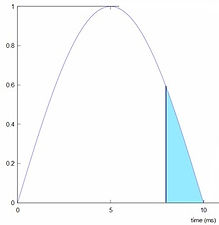# PEMF Pulse windowsSquare gated sine wave carrier frequency? Sounds complicated right? PEMF pulses are applied in pulses per second.

In the above pictures is an example of 3 pulses per second = 3 Hz.

Some devices do just that: giving 3 pulses, which can be simple square waves (as in the picture) or triangle waves or sinus waves. On page 'Pulse forms' I already elaborated on pulse forms and concluded that sinus waves are preferable but how can we obtain a fast 'Speed of induction' (= slew rate) if we use a sine wave? Inherent to the form of a sine wave it can never match the square wave, right?

And here comes the square gated pulse window: Open a square wave 'time window' during which sine waves will pass. Now look at the same picture above and imagine that the orange area's are actually filled with sine waves! The time window simply opens a 'gate' through which these sine waves pass while the 'gate' is open and the gate is only open during the orange time! The sine waves are the actual energy carrier waves because they contain the pulsed electromagnetic energy.

BTW the above square time window must be controlled by a very fast electronic switch to obtain the fast "slew rate".OK but now the next question is: "But the sine waves inside these orange area's are still sine waves with slow rise times so where is the fast 'speed of induction'?"

In this picture you see a (half) sine wave. Notice that the actual sine wave starts at the left bottom corner but only when the maximum is reached the energy is allowed to pass though the gate towards the coils in the applicator, which is the blue area.It is not difficult to see now that the speed of induction

(= slew rate) is superb because this is where the blue area starts!

So what happens now if we do not want to utilize the maximum intensity the system is able to generate but we still want the fast speed of induction, right?

If you look at the picture on the right you see how we do that: we 'simply' let the energy pass at a later stage during the sine wave and we still have the superb slew rate where theblue area starts.